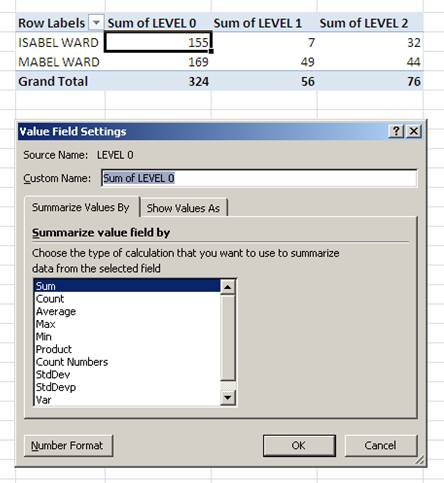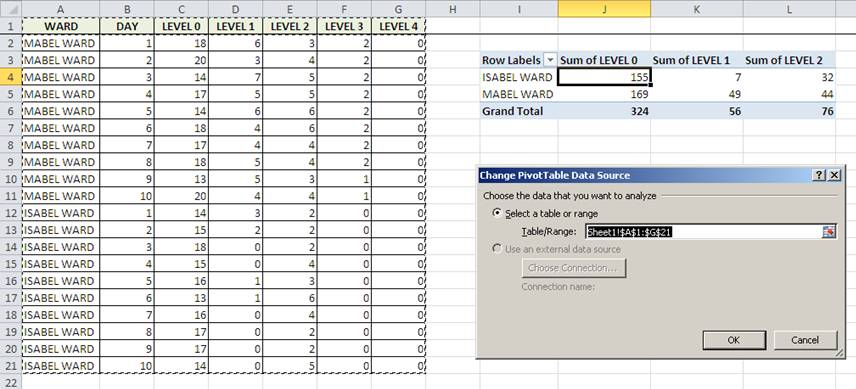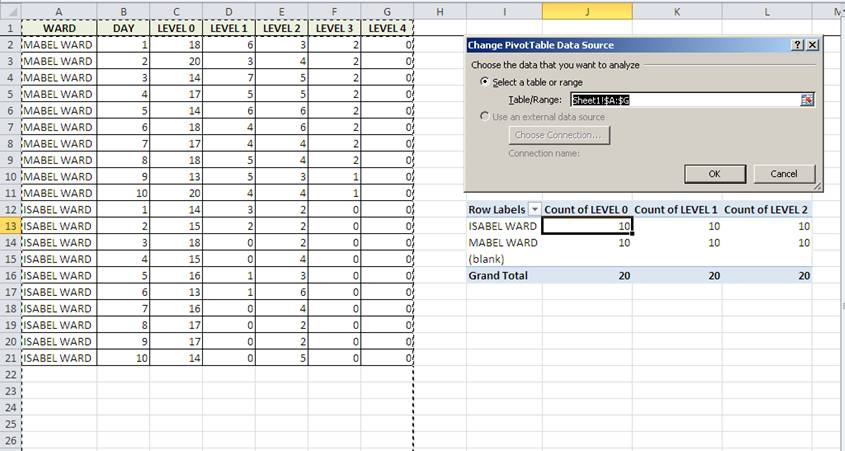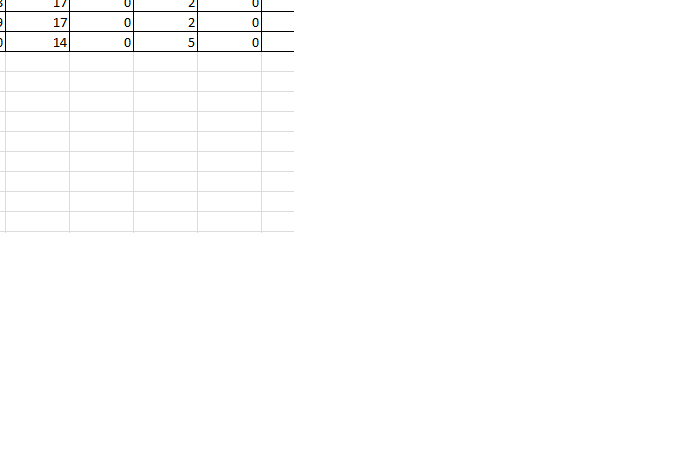The Pivot table is an incredibly powerful tool for summarising data. Excel will either default to summing or counting the field data but you can choose from 11 different functions that include min, max and StdDev as well as the more common Sum, count and Average.

## Why does Microsoft Excel Sum Data instead of Counting data?

If the data you are pivoting contains only numeric data then Excel will sum the data. If there are any blank cells or text cells (other than the header), then Excel will count the data.

I quite often end up with blank cells in my pivot table data. For convenience, I will regularly base my pivot table on a data range that is much larger than the populated data range. I do this so that additional rows of data can be added without having to update the range that the pivot table is looking at. (It is much better to create dynamic pivot table ranges though.)

In the first example, my pivot table is pulling from the populated range and all my fields default to the sum function.In the second example, I have based my pivot table on the columns A:G. This includes many thousands of blank rows and as a result all the fields default to the Count function.## How to change the Summary Calculation in a pivot table

To force Excel to use the Sum function instead of Count, right-click a pivot table cell in the column you wish to change. Choose Summarize Values By and then tick Sum.You can use the same method to select any of the other summary functions.

## Can you change the summary calculation of multiple pivot table fields at once?

Unfortunately there isn’t an easy way to change multiple fields to the same function and right-clicking on a load of data fields gets boring very quickly. If you find yourself having to do this on a regular basis it is worth setting up a macro in your personal workbook so that you can call upon it to very easily change the summary function of every data field in your pivot table.

Here is a short macro that converts all the fields in a selected pivot table to the Sum function. Make sure you have selected the pivot table to change before playing the macro.

``````Public Sub PivotFieldsToSum()
' Cycles through all pivot data fields and sets to sum
' Created by Dr Moxie

Dim pf As PivotField
With Selection.PivotTable
.ManualUpdate = True
For Each pf In .DataFields
With pf
.Function = xlSum
.NumberFormat = "#,##0"
End With
Next pf
.ManualUpdate = False
End With
End Sub
``````

As requested by a commenter, here’s the macro again with the option for the user to select the type of summary function. It isn’t the neatest option as I struggled to pass the user input directly such as `.Function = SubTotalType`

``````Public Sub PivotFieldsToSumUserInput()
' Cycles through all pivot data fields and sets to sum
' Created by Dr Moxie
Dim SubTotalType As String
Dim pf As PivotField

'Add a user input box with default value xlSum
SubTotalType = InputBox("What type of summary do you want? Options are: xlSum, xlAverage, xlCount, xlMax, xlMin", "Summary Type", "xlSum")

With Selection.PivotTable
.ManualUpdate = True
For Each pf In .DataFields
With pf
'.Function = xlSum

'Cycle through predefined summary types
If SubTotalType = "xlMin" Then
.Function = xlMin
ElseIf SubTotalType = "xlCount" Then
.Function = xlCount
ElseIf SubTotalType = "xlAverage" Then
.Function = xlAverage
ElseIf SubTotalType = "xlMax" Then
.Function = xlMax
Else
.Function = xlSum
End If

.NumberFormat = "#,##0"
End With
Next pf
.ManualUpdate = False
End With
End Sub
``````